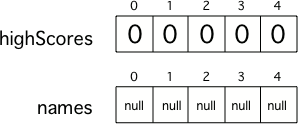# 8.14. Arrays - Summary¶

In this chapter you learned about Arrays. An array is consecutive storage for multiple items of the same type like the top five scores in a game. You learned how to declare arrays, create them, and access array elements. Array elements are accessed using an index. The first element in an array is at index 0.Figure 1: Two 5 element arrays with their values set to the default values for integer and object arrays.

## 8.14.1. Concept Summary¶

• Array - An array can hold many items (elements) of the same type. You can access an item (element) at an index and set an item (element) at an index.

• Array Declaration - To declare an array specify the type of elements that will be stored in the array, then (`[]`) to show that it is an array of that type, then at least one space, and then a name for the array. Examples: `int[] highScores;` `String[] names;`

• Array Creation - To create an array type the name and an equals sign then use the new keyword, followed by a space, then the type, and then in square brackets the size of the array (the number of elements it can hold). Example: `names = new String;`

• Array Index - You can access and set values in an array using an index. The first element in an array called `arr` is at index 0 `arr`. The last element in an array is at the length minus one - `arr[arr.length - 1]`.

• Array Initialization - You can also initialize (set) the values in the array when you create it. In this case you don’t need to specify the size of the array, it will be determined from the number of values that you specify. Example: `int[] highScores = {99,98,98,88,68};`

• Array Length - The length of an array is the number of elements it can hold. Use the public `length` field to get the length of the array. Example: given `int[] scores = {1,2,2,1,3,1};`, `scores.length` equals 6.

• Class Method - A method that can be called on the class. It is declared using the `static` keyword. An example is `Math.abs(-3)`.

• Element Reference - A specific element can be referenced by using the name of the array and the element’s index in square brackets. Example: `scores` will return the 4th element (since index starts at 0, not 1). To reference the last element in an array, use `array[array.length - 1]`

• For-each Loop - Used to loop through all elements of an array. Each time through the loop the loop variable will be the next element in the array starting with the element at index 0, then index 1, then index 2, etc.

• Out of Bounds Exception - An error that means that you tried to access an element of the array that doesn’t exist maybe by doing `arr[arr.length]`. The first valid indices is 0 and the last is the length minus one.

## 8.14.2. Java Keyword Summary¶

• for - starts both a general for loop and a for-each loop. The syntax for a for each loop is `for (type variable : array)`. Each time through the loop the variable will take on the next value in the array. The first time through the loop it will hold the value at index 0, then the value at index 1, then the value at index 2, etc.

• static - used to create a class method, which is a method that can be called using the class name like `Math.abs(-3)`.

## 8.14.3. Practice¶

7-20-1: Drag the item from the left and drop it on its corresponding answer on the right. Click the "Check Me" button to see if you are correct. Review the summaries above.
• The index of the last element
• length - 1
• The number of elements in the array
• length
• The index of the first element
• 0
• The index of the second element
• 1
7-20-2: Drag the description from the left and drop it on the correct code on the right. Click the "Check Me" button to see if you are correct. Review the summaries above.
• Declare an integer array named nums
• int[] nums;
• Declare and create a String array named list1 that can hold 3 elements
• String[] list1 = new String;
• Initialize an array of integers named nums to contain the first 3 whole numbers
• int[] nums = {1,2,3};
• Initialize a String array named list1 to contain the first 3 letters of the alphabet as separate strings
• String[] list1 = {"a", "b", "c"};